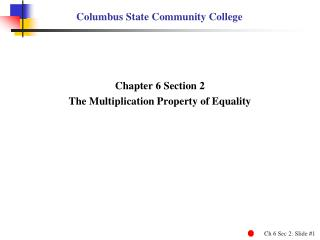Download PresentationColumbus State Community College

# Columbus State Community College - PowerPoint PPT PresentationDownload Presentation## Columbus State Community College

- - - - - - - - - - - - - - - - - - - - - - - - - - - E N D - - - - - - - - - - - - - - - - - - - - - - - - - - -
##### Presentation Transcript

1. Columbus State Community College Chapter 6 Section 2 The Multiplication Property of Equality

2. The Multiplication Property of Equality • Use the multiplication property of equality. • Simplify equations, and then use the multiplication property of equality.

3. Multiplication Property of Equality Multiplication Property of Equality If a,b,c represent real numbers ( c ≠ 0 ), then the equations a = b and a c = b c are equivalent equations. In other words, we can multiply each side of an equation by the same nonzero number without changing the solution.

4. 18x = 24 = 18x = 18 4 18x = 3 Dividing Each Side of an Equation by a Nonzero Number EXAMPLE 1 Dividing by a Nonzero Number Solve 18x = 24. 18x = 24 4 3

5. 4 18 3 1 Dividing Each Side of an Equation by a Nonzero Number EXAMPLE 1 Dividing by a Nonzero Number Solve 18x = 24. Check 18x = 24 6 = 24 1 24 = 24 Balances

6. 3.2 m 24.96 = 3.2 3.2 Solving an Equation with Decimals EXAMPLE 2 Solving an Equation with Decimals Solve 3.2 m = 24.96 . 3.2 m = 24.96 m = 7.8

7. Solving an Equation with Decimals EXAMPLE 2 Solving an Equation with Decimals Solve 3.2 m = 24.96 . Check 3.2 m = 24.96 3.2 ( 7.8 ) = 24.96 24.96 = 24.96 Balances

8. 2 = Solve . 30 w 5 5 5 2 w = 30 2 2 2 = 30 w 5 5 w = 75 Using the Multiplication Property of Equality EXAMPLE 3 Using the Multiplication Property of Equality 1 1 15 1 1 1

9. 2 = Solve . Check 30 w 5 2 w = 30 5 2 = 30 ( 75 ) 5 = 30 Using the Multiplication Property of Equality EXAMPLE 3 Using the Multiplication Property of Equality 30 Balances

10. –1 h 15 = –1 –1 Coefficient of the Variable is –1 EXAMPLE 4 Using the Multiplication Property of Equality Solve –h = 15. Method 1 Method 2 –h = 15 – h = 15 –1h = 15 –1h = 15 –1 ( –1 h ) = –1 ( 15 ) h = –15 h = –15

11. Coefficient of the Variable is –1 EXAMPLE 4 Using the Multiplication Property of Equality Solve –h = 15. Check –h = 15 –1 ( –15 ) = 15 15 = 15 Balances

12. The Multiplication Property of Equality Chapter 6 Section 2 – Completed Written by John T. Wallace